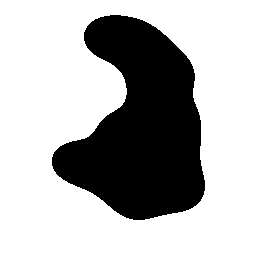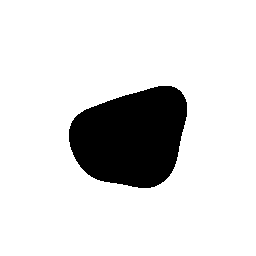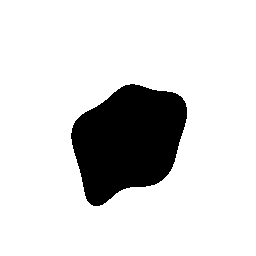## RANDOMBLOB

 Creates an image composed of a moderate sized black random blob shape on a white background.

 Variation In Seed Uniform Distribution On Square: Seed=1 Arguments: -o 256 -i 200 -s square -k uniform -n 12 -l 7 -b 16 -g 33 -S 1 Uniform Distribution On Square: Seed=1003 Arguments: -o 256 -i 200 -s square -k uniform -n 12 -l 7 -b 16 -g 33 -S 1003Uniform Distribution On Disk: Seed=1 Arguments: -o 256 -i 200 -s disk -k uniform -n 12 -l 7 -b 16 -g 33 -S 1 Uniform Distribution On Disk: Seed=1003 Arguments: -o 256 -i 200 -s disk -k uniform -n 12 -l 7 -b 16 -g 33 -S 1003Gaussian Distribution On Square: Seed=1 Arguments: -o 256 -i 200 -s square -k gaussian -n 12 -l 7 -b 16 -g 33 -S 1 Gaussian Distribution On Square: Seed=1003 Arguments: -o 256 -i 200 -s square -k gaussian -n 12 -l 7 -b 16 -g 33 -S 1003Gaussian Distribution On Disk: Seed=1 Arguments: -o 256 -i 200 -s disk -k gaussian -n 12 -l 7 -b 16 -g 33 -S 1 Gaussian Distribution On Disk: Seed=1003 Arguments: -o 256 -i 200 -s disk -k gaussian -n 12 -l 7 -b 16 -g 33 -S 1003Variation In Number Of Points Uniform Distribution On Square: 12 Points Arguments: -o 256 -i 200 -s square -k uniform -n 12 -l 7 -b 16 -g 33 -S 1 Uniform Distribution On Square: 16 Points Arguments: -o 256 -i 200 -s square -k uniform -n 16 -l 7 -b 16 -g 33 -S 1Uniform Distribution On Disk: 12 Points Arguments: -o 256 -i 200 -s disk -k uniform -n 12 -l 7 -b 16 -g 33 -S 1 Uniform Distribution On Disk: 16 Points Arguments: -o 256 -i 200 -s disk -k uniform -n 16 -l 7 -b 16 -g 33 -S 1Gaussian Distribution On Square: 12 Point Arguments: -o 256 -i 200 -s square -k gaussian -n 12 -l 7 -b 16 -g 33 -S 1 Gaussian Distribution On Square: 16 Points Arguments: -o 256 -i 200 -s square -k gaussian -n 16 -l 7 -b 16 -g 33 -S 1Gaussian Distribution On Disk: 12 Points Arguments: -o 256 -i 200 -s disk -k gaussian -n 12 -l 7 -b 16 -g 33 -S 1 Gaussian Distribution On Disk: 16 Points Arguments: -o 256 -i 200 -s disk -k gaussian -n 16 -l 7 -b 16 -g 33 -S 1